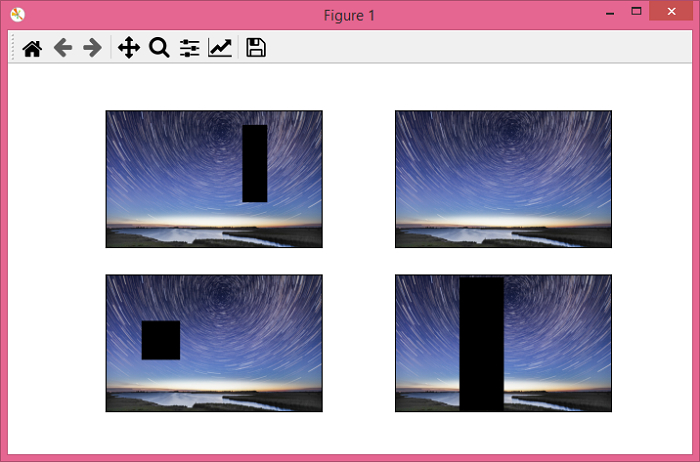# PyTorch – torchvision.transforms – RandomErasing()

PyTorchServer Side ProgrammingProgramming

The RandomErasing() transform randomly selects a rectangular region in an input image and erases its pixels. The torchvision.transforms module provides many important transforms that can be used to perform different types of manipulations on the image data. RandomErasing() transformation accepts only tensor images of any size. A tensor image is a torch tensor.

As this transform supports only tensor image, the PIL images should be first converted to a torch tensor. And after applying the RandomErasing() transform, we convert torch tensor image to PIL image.

## Steps

We could use the following steps to randomly select a rectangular region in an input image and erase its pixels −

• Import the required libraries. In all the following examples, the required Python libraries are torch, Pillow, and torchvision. Make sure you have already installed them.

import torch
import torchvision
import torchvision.transforms as T
from PIL import Image
• Read the input image. The input image is a PIL image or a torch tensor.

img = Image.open('sky.jpg')
• If the input image is PIL image, convert it to a torch tensor.

imgTensor = T.ToTensor()(img)
• Define RandomErasing() transform.

transform = T.RandomErasing(p=0.5, scale=(0.02, 0.33), ratio=(0.3, 3.3), value=0, inplace=False)
• Apply the above-defined transform on the input image to randomly select a rectangular region in an input image and erase its pixels.

imgTensor = transform(imgTensor)
• Convert the above-transformed tensor image to PIL image.

img = T.ToPILImage()imgTensor)
• Show the normalized image.

img.show()

### Note

Alternatively, we can define a composition of the above three transformations performed in 3rd, 4th and 6th steps.

transform = T.Compose([
T.ToTensor(),
T.RandomErasing(p=1, scale=(0.02, 0.33), ratio=(0.3, 3.3), value=0, inplace=False),
T.ToPILImage()
])



## Input Image

This image is used as the input file in all the following examples.## Example 1

The following program randomly selects a rectangular region from the input image and erases its pixels. Here, we have set the probability to 1, so it will definitely select a region in the image and erase its pixels.

# import required libraries
import torch
import torchvision.transforms as T
from PIL import Image

img = Image.open('sky.jpg')

# define a transform to perform three transformations:
# convert PIL image to tensor
# randomly select a rectangle region in a torch Tensor image
# and erase its pixels
# convert the tensor to PIL image
transform = T.Compose([ T.ToTensor(), T.RandomErasing(p=1, scale=(0.02, 0.33), ratio=(0.3, 3.3), value=0, inplace=False), T.ToPILImage() ])
# apply the transform on image
img = transform(img)

# display the output image
img.show()

## Output

It will produce the following output −## Example 2

Let's take another example −

import torch
import torchvision.transforms as T
from PIL import Image
import matplotlib.pyplot as plt

img = Image.open('sky.jpg')

# define a transform to perform transformations
transform = T.Compose([T.ToTensor(), T.RandomErasing(p=0.5, scale=(0.02, 0.33), ratio=(0.3, 3.3), value=0, inplace=False), T.ToPILImage()])

# use dictionary comprehension to take four output images
imgs = [transform(img) for _ in range(4)]

# display four output images
fig = plt.figure(figsize=(7,4))
rows, cols = 2,2
for j in range(0, len(imgs)):
plt.imshow(imgs[j])
plt.xticks([])
plt.yticks([])
plt.show()

## Output

It will produce the following output −Notice that in the above output images, at least two out of the four images have erased regions, as we have set the probability to 0.5.